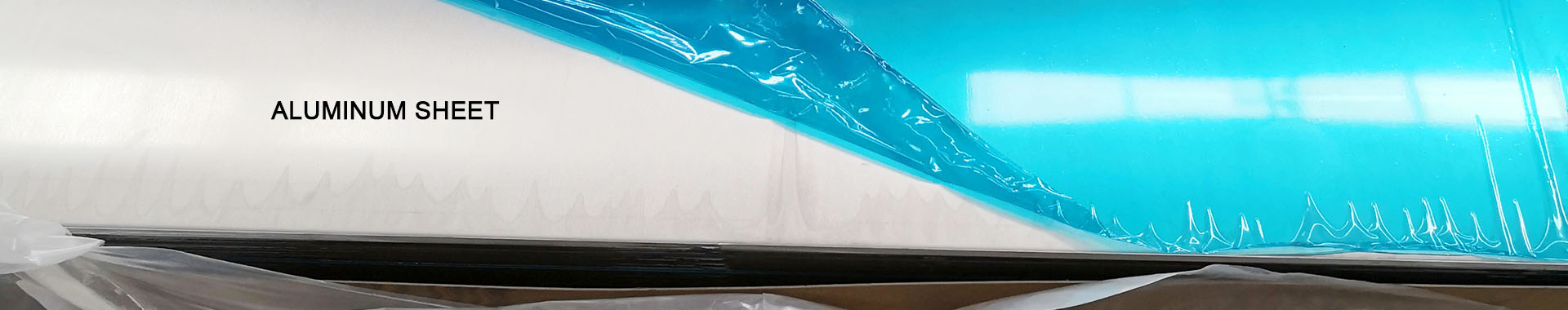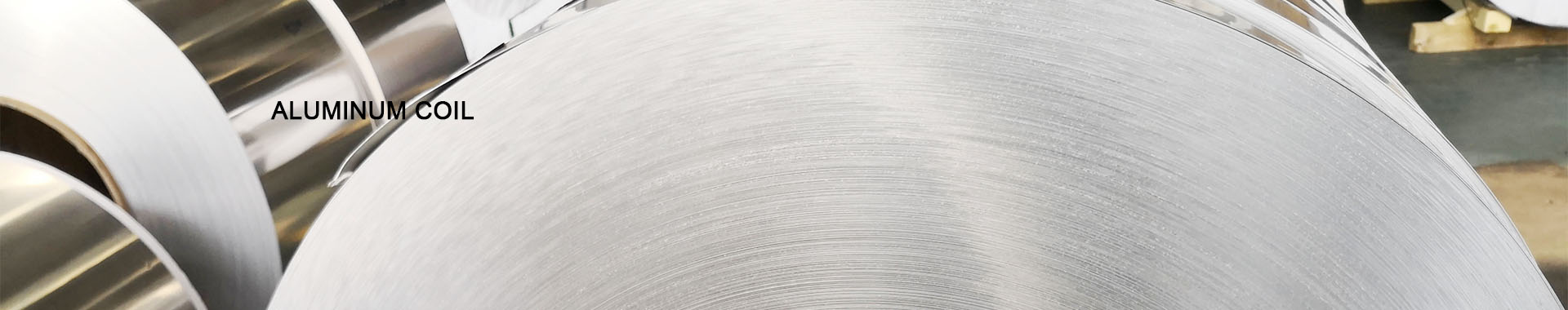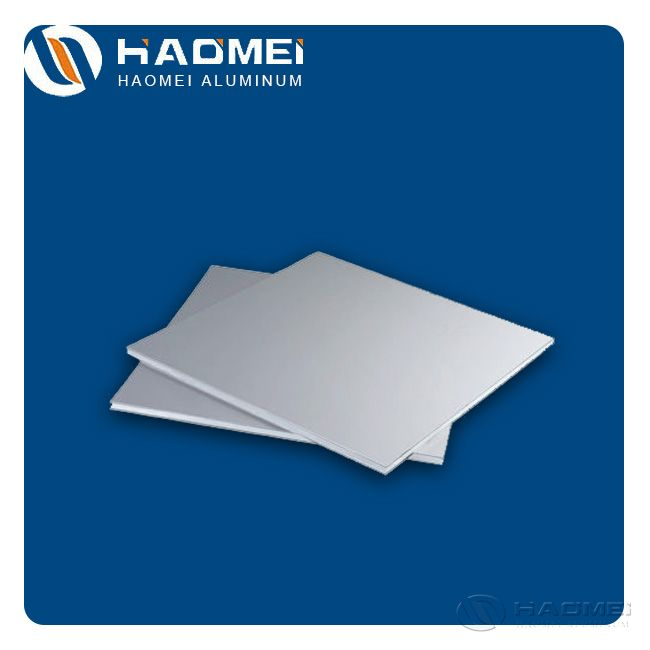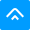Get Quot：

sale@alumhm.com
•••Home» Blog» 3mm Aluminium Sheet Weight

# 3mm Aluminium Sheet Weight

Publish: Modify: Jul 22, 2020Author: From:  HaomeiThe formula for calculating 3mm aluminium sheet weight is very simple. We need to know the 3mm aluminium sheet sizes and the density. There is an example of 1050 aluminum.

3mm aluminum sheet is a common specification. Many customers always ask about what’s the 3mm aluminium sheet weight? In fact, it is easy to calculate.The 3 mm aluminium sheet sizes multiplied by density is the weight. The aluminum sheet sizes result is got by multiplying the length, the thickness and the width. The density of aluminum plates varies according to the metal content.

The density of aluminum plates of 1-8 series is between 2.71-2.75. Assuming the density of 2.73 is calculated, theoretically a piece of 3mm*1000mm*1000mm weighs 8.19kg. And if the aluminum plates of this specification are relatively flat and stacked together, the estimated number of sheets per cubic meter should be 300-333. So the theoretical weight should be about two and a half tons per one cubic meter.

Let’s come to have a look of the specific calculating steps:

1. Volume= length * width * thickness

2. Weight= density*volume

As we have mentioned, the density of different aluminum alloys sheets is different. There are some common alloys such as 1060, 3003 and 6061. The density of 1060 aluminum sheet is 2.71 g/cm3, that of 3003 aluminum sheet is 2.72 g/cm3and that of 6061 aluminum alloy is 2.71g/cm3. What’s the weight of 3 mm 1050 aluminum sheet with length of 1000mm and 800 mm? The volume of aluminum sheet is 2400 cm. The weight=2.71*2400 =6504g.

### Inquiry

 Name: Email: Tel/Whatsapp: Country: Message: SubmitHaomei Aluminum CO., LTD.

Tel/Whatsapp: +86-15978414719

Email: sale@alumhm.com

Website: https://www.alumhm.com

Xin'an Industrial Assemble Region,Luoyang,Henan Province,China